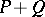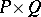# Disjoint sum of partially ordered sets

(diff) ← Older revision | Latest revision (diff) | Newer revision → (diff)
Letandbe two partially ordered sets (cf. Partially ordered set).
The disjoint sumofandis the disjoint union of the setsandwith the original ordering onandand no other comparable pairs. A poset is disconnected if it is (isomorphic to) the disjoint sum of two sub-posets. Otherwise it is connected. The maximal connected sub-posets are called components.
The disjoint sum is the direct sum in the category of posets and order-preserving mappings. The direct product in this category is the Cartesian productwith partial ordering